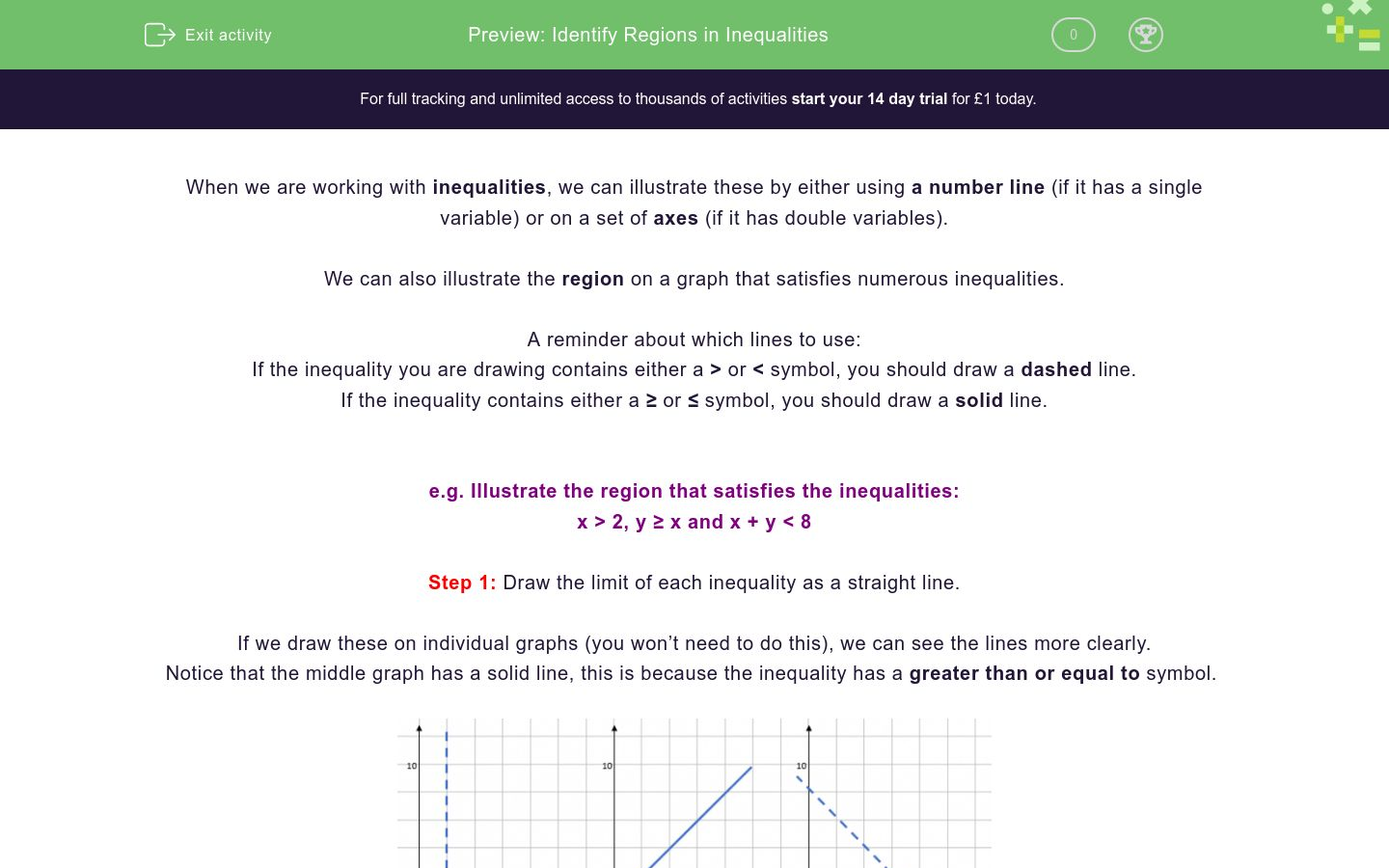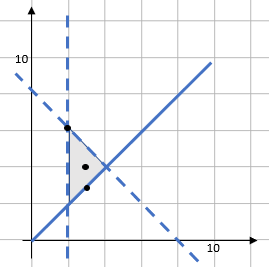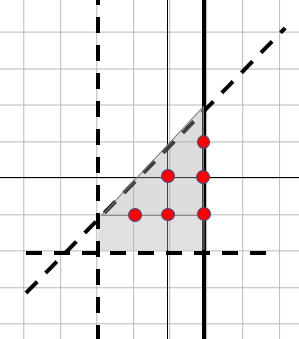# Identify Regions in Inequalities

In this worksheet, students will practise identifying the region defined by inequalities.Key stage:  KS 4

GCSE Subjects:   Maths

GCSE Boards:   Eduqas, OCR, AQA, Pearson Edexcel

Curriculum topic:   Geometry and Measures, Indices and Surds, Number, Algebra

Difficulty level:### QUESTION 1 of 10

When we are dealing with inequalities, we have so far learnt to illustrate these by either using a number line (if it is a single variable) or on a set of axes (if it’s double variable).

What we have to do now is illustrate the region on a graph that satisfies numerous inequalities.

If the inequality you are drawing contains either > or <, you draw a dashed line.

If the inequality contains either ≥ or ≤, you draw a solid line.

Example: Illustrate the region that satisfies the inequalities.

x > 2, y ≥ x and x + y < 8

Step 1: Draw the limit of each inequality as a straight line.

If we draw these on individual graphs (you won’t need to do this in an exam), we can see the lines.

Notice that the middle graph has a solid line, this is because the inequality is greater than or equal to.We would actually draw all of these onto one graph.Step 2: Illustrate the region that satisfies all three inequalities.

There’s a short cut to this, in all my time teaching, I’ve never seen it not work at GCSE (it’s slightly different at A-Level)

Find the section that is defined by the inequalities. There is only one bit on here that works for all the inequalities, the bit in the middle.Example: Are these points in the region defined by the inequalities x > 2, y ≥ x and x + y < 8

1. (3,4)
2. (2,6)
3. (3,3)

If we plot these on the graph we previously created.1. (3,4) – This is in the middle of the region so it’s fine
2. (2,6) – This is on a dashed line. Because a dashed line means > or <, points on the line don’t satisfy
3. (3,3) – This point is on a solid line. A solid line means ≥ or ≤. Points on these lines do satisfy.

When we are finding the region for multiply inequalities, we shade the area that...

We use a dashed line for inequalities containing...

Which of these graphs show the correct lines for the equations

y = - 4, x = 2 and y = 2x + 1Left Hand graph

Right Hand graph

Which region satisfies the inequalities

y > - 4, x < 2 and y < 2x + 1A

B

C

I want to draw the inequalities

-2 < x ≤ 1, y > -2 and  y < x + 1

In which of these two diagrams have the inequalities been drawn correctly?A

B

How many integer points are there that satisfy the following inequalities?

-2 < x ≤ 1, y > -2 and  y < x + 1

I want to draw the inequalities

3y + 2x > 12, y < x – 1 and x < 6.

Which one of these two graphs is correct?A

B

Do the following points satisfy all three inequalities.

3y + 2x > 12, y < x – 1 and x < 6.

Which of the following two diagrams shows the inequalities

X &ge 2, y > x and x + y ≤ 6 correctly.A

B

Select the points that do satisfy all three equations.

X &ge; 2, y > x and x + y ≤ 6

• Question 1

When we are finding the region for multiply inequalities, we shade the area that...

EDDIE SAYS
You will sometimes see examples where they shade the area that doesn’t satisfy each inequality. This allow the region that satisfies to be left blank. This is a better approach if you are doing each one individually, but not when you are using the shorter method I described.
• Question 2

We use a dashed line for inequalities containing...

EDDIE SAYS
We need to differentiate between > and ≥. As a point that is > cannot be on the line, we use dashed line to show this.
• Question 3

Which of these graphs show the correct lines for the equations

y = - 4, x = 2 and y = 2x + 1Left Hand graph
EDDIE SAYS
It’s really important to get your y = a and x = a the right way round. If a line says x = 2, it goes through the x axis at 2, it will be vertically up.
• Question 4

Which region satisfies the inequalities

y > - 4, x < 2 and y < 2x + 1B
EDDIE SAYS
We need to find the points that are defined by all the inequalities. A is only satisfied by 1 of the lines, C is only satisfied by 1 of the lines.
• Question 5

I want to draw the inequalities

-2 < x ≤ 1, y > -2 and  y < x + 1

In which of these two diagrams have the inequalities been drawn correctly?B
EDDIE SAYS
This is about the types of lines that have been drawn. Remember that > or < are dashed lines and ≥ or ≤ are solid lines.
• Question 6

How many integer points are there that satisfy the following inequalities?

-2 < x ≤ 1, y > -2 and  y < x + 1

6
EDDIE SAYS
Integer points means that the values of X and Y must be integers. In an inequality, points on dashed lines don’t satisfy and points on solid lines do. Did you get all six points (shown in red)• Question 7

I want to draw the inequalities

3y + 2x > 12, y < x – 1 and x < 6.

Which one of these two graphs is correct?A
EDDIE SAYS
Once again, its all about the lines. All of the inequalities are > or < so they should all be dashed lines.
• Question 8

Do the following points satisfy all three inequalities.

3y + 2x > 12, y < x – 1 and x < 6.

EDDIE SAYSIf you plot the points, any that are inside the region are fine, any that are outside the region don’t satisfy the inequalities. The point will only satisfy the inequalities if it is on a solid line or in the region.
• Question 9

Which of the following two diagrams shows the inequalities

X &ge 2, y > x and x + y ≤ 6 correctly.A
EDDIE SAYS
Were you looking to see if the lines were correct for dashes or solid? This is going back to that simple mistake of drawing the line incorrectly, which way should x = 2 go?
• Question 10

Select the points that do satisfy all three equations.

X &ge; 2, y > x and x + y ≤ 6

EDDIE SAYSThe point will only satisfy the inequalities if it is on a solid line.
---- OR ----

Sign up for a £1 trial so you can track and measure your child's progress on this activity.

### What is EdPlace?

We're your National Curriculum aligned online education content provider helping each child succeed in English, maths and science from year 1 to GCSE. With an EdPlace account you’ll be able to track and measure progress, helping each child achieve their best. We build confidence and attainment by personalising each child’s learning at a level that suits them.

Get started